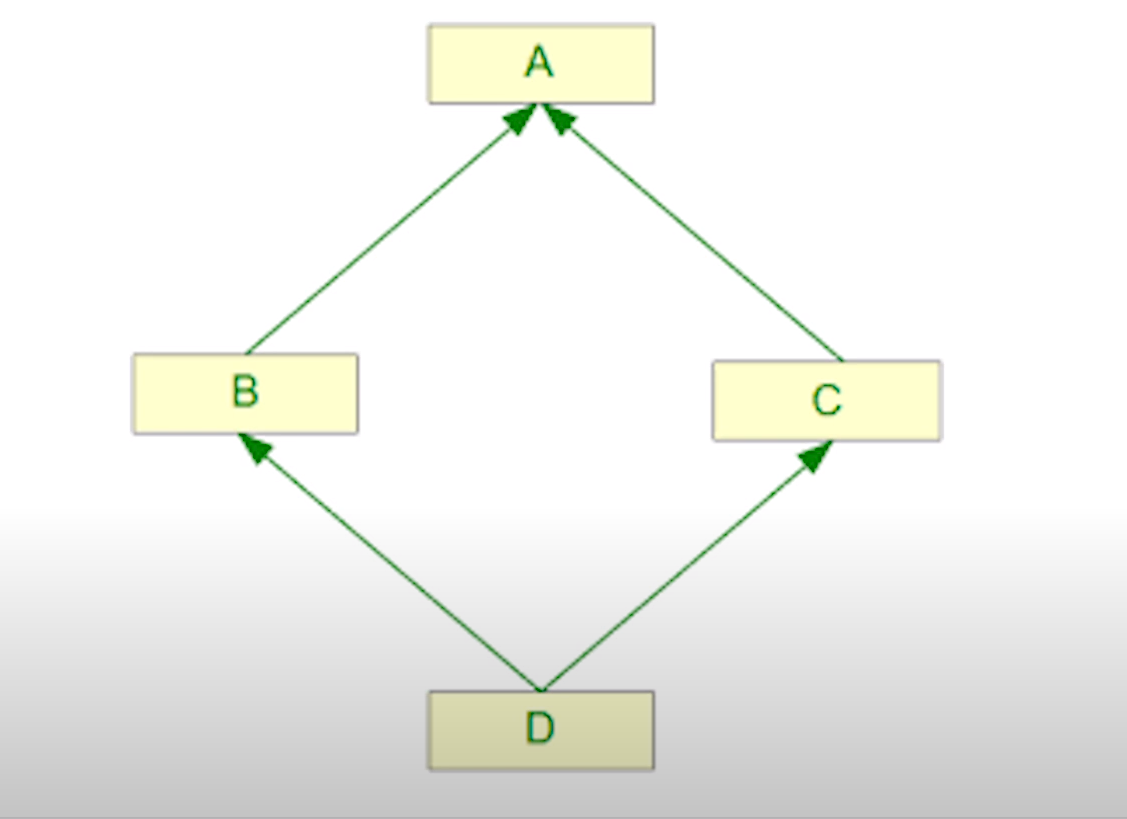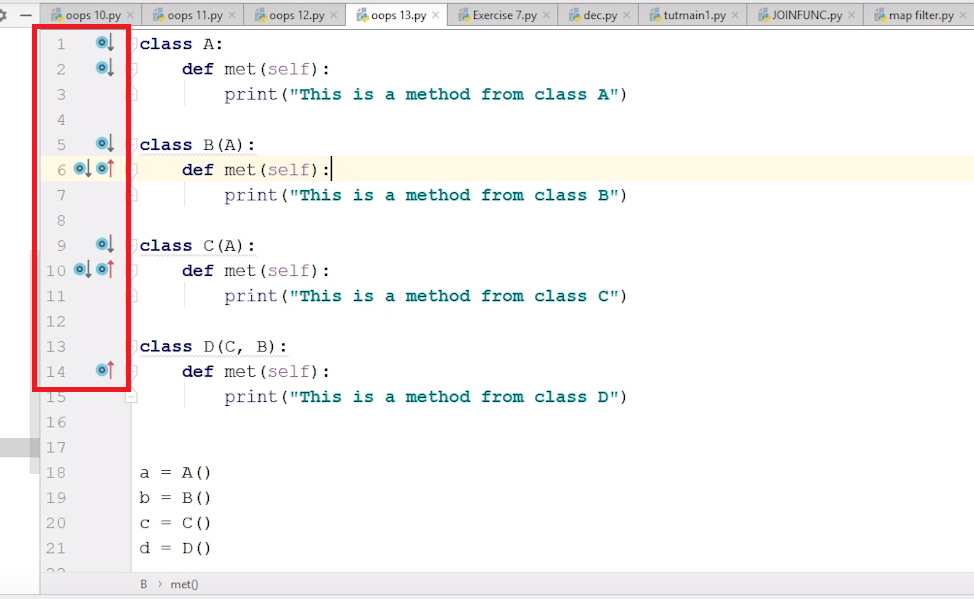### Diamond Shape Problem In Multiple Inheritance | Python Tutorials For Absolute Beginners In Hindi #66

From the start of this course, you may have noticed that I am not just teaching you the syntax so that you may learn just the practical approach to programming and could create a few programs that have no real-world value. Instead, I am trying to teach you all the theoretical and practical concepts together so you may become a successful programmer. You can do the programming by just learning the syntax, but without proper conceptual knowledge, you won't be able to develop proper logic while writing code. Our today’s tutorial is also based on a theoretical concept.

In previous tutorials, we have seen a lot of concepts related to Object-Oriented programming such as Single inheritance, Multiple Inheritance, Multilevel Inheritance, etc. Today we are going to discuss a problem or more like a confusion associated with multiple inheritance. The problem is commonly known as the “Diamond Shape Problem.”. It is about a priority related confusion, which arises when four classes are related to each other by an inheritance relationship, as shown in the image below:#### Explanation:-

Now we can see that the class C and class B are inheriting from class A, or it can be said as, class A is a parent to class B and C. And class D is inheriting from both class C and B. So, in a way they are all in relation to one and other somehow. Let us write down the relation in code format so it will be easier to understand.

``````class A:
pass
class B(A):
pass
class C(A):
pass
class D( B, C ):
pass``````

As discussed earlier that it creates a priority related confusion, so lets clear that out here.

• If we have a method that is only present in class A and we use class D object to call the method, it will go to class A while checking for the method name in all the classes in between and run the method in class A.
• However, if the same method is also present in class B, then it will run the B class method because for class D, class B holds a more priority then class A. The reason is that class D is derived from class B which is further derived from A. So, the closer relation exists with B than A.
• If the same method is present in class C and B, it may create a little bit of confusion. But as we have already discussed in Tutorial #61, that in such cases, our priority is based from left to right, meaning whichever class is on the left side will be given more priority, and then we will move towards right one. In this case, the left class is B, so the method in B will de be executed first.

If the C class would be on left, such as

`````` class D( C,B ):
pass``````

Then priority would be given to C.

Sometimes, when we are working with too many classes, the concept of multiple inheritance could make our code more confusing and difficult to understand, such as:You can see in the highlighted area that, it is a little difficult to understand which method is overriding which one, so multiple inheritance is discouraged in such situations.

#### Code as described/written in the video

``````
class A:
def met(self):
print("This is a method from class A")

class B(A):
def met(self):
print("This is a method from class B")

class C(A):
def met(self):
print("This is a method from class C")

class D(C, B):
def met(self):
print("This is a method from class D")

a = A()
b = B()
c = C()
d = D()

d.met()

``````